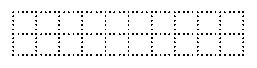Technology for Artists

A discussion of ... uh, technology for artists. Duh!

Monday, September 10, 2007

Math Without Math: Functions

One of the cool things about Cartesian coordinates is that we can describe pictures with numbers. This means that we can use computers for what they do best ... computing ... in helping to create and display pictures. One category of mathematical tools that we use for this is functions. Functions also show up in programming, with a similar meaning, so they're good things to know about.

A function is basically a rule for combining one or more numbers to get another number. For example, we can think of the average temperature in a city as a function of the month. For a Northern climate, the high temperature will be highest in the Summer months, lowest in the Winter months, and in between in the Fall and Spring. There will be some wiggling around because temperatures don't follow a hard and fast rule.

We can use Cartesian coordinates to visualize a function. We have two numbers: the month of the year, and the average temperature for that month. Whenever we have two related numbers like this, we can use them to indicate points on a graph. For the temperature, the graph might look something like this:This is really two functions: the high average temperature and the low average temperature in New York City for each month.\footnote{Source: New York City: Average Temperatures (http://www.ny.com/general/weather.html) Both of them rise and fall with the seasons, but the high temperature is ... um, higher.

Squaring a number is another example of a function. We can make a graph of numbers and their squares. It looks like this:The reverse of this, the square root, is also a function we can graph:Notice that the square root function looks like the square function flipped over on it's side. That's because it is. Remember that if you have two numbers, and one is the square of the other, than the other is the square root of the first. So we're really just switching the horizontal and vertical directions of the graph.

Sometimes a function will take two numbers and give you a third. For example, if you scan a piece of art at some resolution, the size of the file is a function of the width and height of the art. Change either one of those and you change the file size.. This is called a multi-variate function, since there are two variables. The function is area = width x length.

Since there are three numbers, the two variables and the result, we can't graph this function in 2 dimensions. We need to use 3 dimensions. The graph would look something like this:Who says math is not visual?

So what the heck does this mean? Suppose your art is only 1 inch tall, and so many inches wide.Then making it an inch longer adds just one extra square inch. If we scan at 300 samples per inch, then 1 square inch is about 270,000 bytes. That's because each sample is 3 bytes, and one inch has 300 of those samples. That's 900 bytes just for a one pixel wide scan. But a square in is 300 pixels wide, so we multiply again: 3 x 300 x 300 = 270,000.

However, if the picture were 2 inches tallthen each inch wider adds 2 square inches. So that means that as the width increases, the 2 inch tall picture grows twice as fast as the one inch tall one. A 3 inch tall picturewould grow three times as fast. We can graph this like this:Here the red line is the function that gives the file size of a 1 inch tall picture for different widths of the picture. The blue line gives the same function for a 2 inch tall picture, and the green line is for a 3 inch tall picture. As you can imagine, we could keep on for a 4 inch tall picture, 5 inches, and so on, and the lines would just keep getting steeper. So the taller the picture is, the more effect changing the width has, and vice versa.

We could graph this in 3D like this:We made all the lines blue, but each blue line still represents one height of the scanned picture, at different widths. The first blue line, closest to the front, is for pictures 1 inch tall. At the left end, where the width is very small, the overall size of the scanned file will be small. Towards the right end of that line, the width is larger, so the size of the scanned image file will be larger. The line behind that is for 2 inch tall scans, so it's steeper. Behind that is 3 inches, etc. So the taller the picture is, the more a small change in width will affect it.